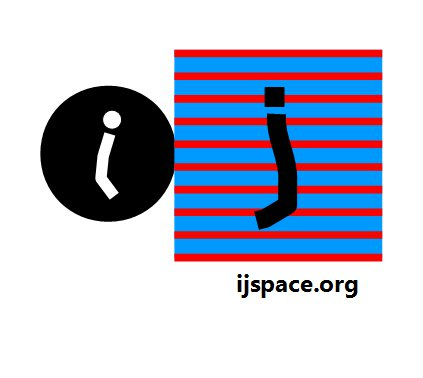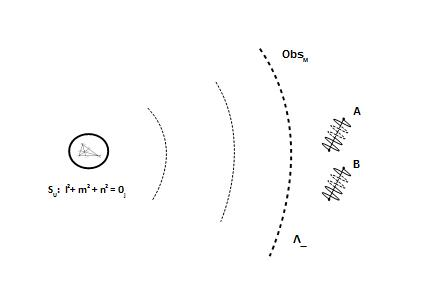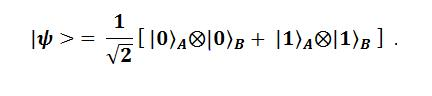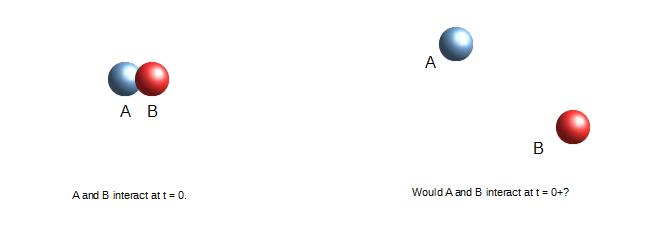# Home

 EPR  Paradox-II 23rd July 2016 "We should not forget that only a small portion of the world is known with accuracy." - Charles Darwin, On the Origin of Species by Means of Natural Selection.     "The requirements was, and is, to obtain the most profitable result from imperfectly postulated data; and the data may possibly be subjected to conditions, which likewise are imperfectly postulated." - A. R. Forsyth, Calculus of Variations. "Tell me the environment, and I'll tell you the probability after a next moment of time that this point is at state s. And that's the way it's going to work, okay?" - Feynman discussing Quantum Computers. "Indeed, we find ourselves here on the very path taken by Einstein of adapting our modes of perception borrowed from the sensations to the gradually deepening knowledge of the laws of Nature. The hindrances met with on this path originate above all in the fact that, so to say, every word in the language refers to our ordinary perception. In the quantum theory we meet this difficulty at once in the question of the inevitability of the feature of irrationality characterising the quantum postulate. I hope, however, that the idea of complementarity is suited to characterise the situation, which bears a deep-going analogy to the general difficulty in the formation of human ideas, inherent in the distinction between subject and object." - Neils Bohr, Como Lecture, 1928.For the structures  A and B as shown in the diagram, the quantum entanglement condition can be defined by the following wave-function1:Above relationship represents two structures A and B which are not independent of each other.  If the structure A is measured as in state |0〉 then the structure B is simultaneously measured as in state |0〉. Similar argument holds for the state |1〉. The important point is to note that Locality condition holds for the structures A, B and the entangled state, i.e. the communication between the structures A and B is restricted to the speed of light. Which in turn means that the structures A and B and the entangled state, belong to the same measurement space. Please note that the Locality condition is implicit in VT-symmetry which defines the origin in measurement space. The maximum measurement capacity possible is v/c ~1.  The measurement themselves are being performed by the macroscopic observer ObsM with capacity v/c << 1. In the discrete measurement space (j-space), the states |0〉 and |1〉 correspond to the measured values and they are measured by ObsM.       The measurements corresponding to the state |0〉 as performed by ObsM, when evaluated under Obsi (v/c >> 1) criterion, will be measured as the state |0j〉 which is a finite value state. In fact Obsi will measure {.......|0j〉.....|1j〉..........} , which contains enormously more information, than just  {|0j〉, |1j〉} as measured by ObsM. Thus Obsi, will be able to measure all the states possible between |0j〉 and |1j〉  and beyond, which is the essential classical scenario. The classical scenario implies that there is no uncertainty in measurements when made in the observer's measurement metric and the observer has enough resources so that the observer itself, can behave as a source to initiate time axis for the structures under measurements. The wave-function is represented by a delta function (no uncertainty), in this case.     We are trying to determine whether in j-space the behaviour of two structures is influenced by each other, if they had interacted with each other at an earlier instant. The situation is described in the following diagram:Each observer is allowed to measure with arbitrary precision within his measurement metric. Important to note is that the arbitrary precision does not imply infinite precision. Arbitrary precision is equivalent to the maximum capacity of the observer making the measurement. Consider the situation shown above for t = 0+ as measured by two observers with different capacities. One of the observer is ObsM and other observer is Obsi. We have to remember that the measurements made by ObsM can not resolve what the measurements made by Obsi can. Both cases are shown below:For ObsM the measurements will not detect the underlying relationship between Λ∞-plane and structures. And the measurements definitely can not determine the relationship between the Unit-point sphere SU and the structures being measured. Thus in classical case the arbitrary precision available, is not precise enough to measure the influence of the structures A and B on each other when they are sufficiently far apart. However Obsi measurements made with its arbitrary precision will be able to determine the relationship between A, B and the Unit-point sphere SU.     The j-space is the discrete measurement space corresponding to the observer's capacity. In j-space we can not have absolute stillness or structures moving with infinite velocity2. Therefore the structures A and B, even though if they are measured as at rest by ObsM, will not be still. We also need to keep in mind that each structure, A or B, itself can be thought of as observers in j-space. Any movement in j-space is not possible without A and B themselves making measurements, which will result in the interaction with Λ∞-plane.     Furthermore the Λ∞-plane(A) and Λ∞-plane(B) will always intersect each other unless they correspond to different Anharmonic Structures i.e. different ΔOXY's3. Therefore the observer Obsi will evaluate the situation as that, even though the A and B are apart in ObsM measurement metric, A and B are influenced by the behaviour of each other. In fact the requirement that they must have interacted at instant t = 0 in ObsM's (t, x, y, z) measurement metric, is of no consequence.      So what happens if the one of the structures, let us say A, is dropped in to a black-hole? Is an interaction still possible between the structures A and B? The definition of the black-hole is dependent on the observer's capacity. If a black-hole exists per measurements of ObsM, it does not necessarily mean that it will exist for an observer with higher capacity, for example Obsi. In j-space the interaction between structures will always exist whether an observer is capable of measuring it or not.     In our discussion so far, we have used neither the wave-function nor the stochastic nature of Quantum Mechanics while discussing the "entanglement". We have used measurements made by the observer Obsi as the benchmark criterion for determining the interaction between the structures A and B. We note that for the observer Obsi,  the condition v/c >> 1 applies, that means in (t, x, y, z) metric Lorentz Invariance does not hold neither does the locality condition, for the measurements made by Obsi. The phenomenological explanation of the value of the fine-structure constant seems to indicate that we are in q = 3 state in the information space and therefore higher information states, q = (1, 2), are possible. The locality condition does not allow the existence of these higher information states.        We will later argue that the stochastic nature of QM, is due to the fact that we are trying to estimate the mean of a real-valued variable based on a sample size (n), which is much smaller than the actual spectrum    (N → ∞) of the variable. We will try to understand it geometrically. It is also important to resolve this perceived conflict between Special Theory of Relativity and Quantum Mechanics. Both of them are powerful methodologies who at best approximate the physical reality for the macroscopic observer in Λ∞-plane. Both of these theories represent limitations of the observer's capacity, and therefore they should correlate to the definition of origin in j-space i.e. VT-symmetry.     Non-locality vs. Local Hidden Variables? Non-locality! ___________________ 1Note that we have used the inner-product symbol ⨂ instead of the scalar product. The reason being that we wanted to highlight the fact that we can perform addition and hence multiplication only for stable distributions in j-space. Therefore |0〉 and |1〉 states must be represented by one of the stable distributions. The addition of probability distributions is not a standard arithmetic operation.  The conventional arithmetic operations are legitimate only in the measurement metric for the observer ObsM (v/c << 1).2 Being completely still or moving with infinite velocity are equivalent to stating that the observer has an infinite capacity to measure the source. But then we will not be in j-space to begin with. 3 If that was the case then the macroscopic observer ObsM will not be able to measure the structures A and B simultaneously.Previous Blogs: EPR Paradox-I  A Paradox The Observers Nutshell-2015 Chiral Symmetry Sigma-z and I Spin Matrices Rationale behind Irrational Numbers The Ubiquitous z-Axis Majorana ZFC Axioms Set Theory Nutshell-2014 Knots in j-Space Supercolliders Force Riemann Hypothesis Andromeda Nebula Infinite Fulcrum Cauchy and Gaussian Distributions Discrete Space, b-Field & Lower Mass Bound Incompleteness II The Supersymmetry The Cat in Box The Initial State and Symmetries Incompleteness I Discrete Measurement Space The Frog in Well Visual Complex Analysis The Einstein Theory of RelativityBest viewed with Internet Explorer